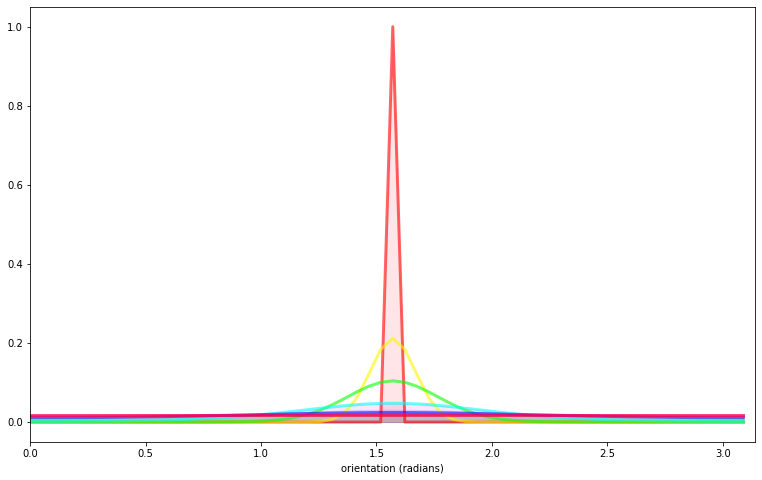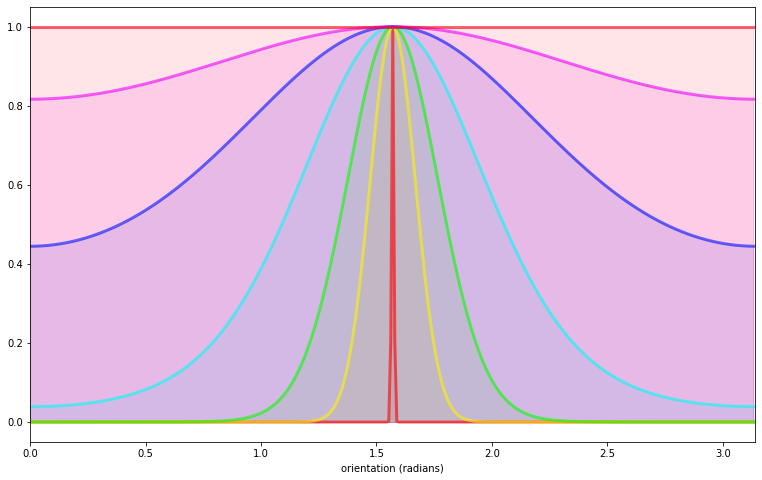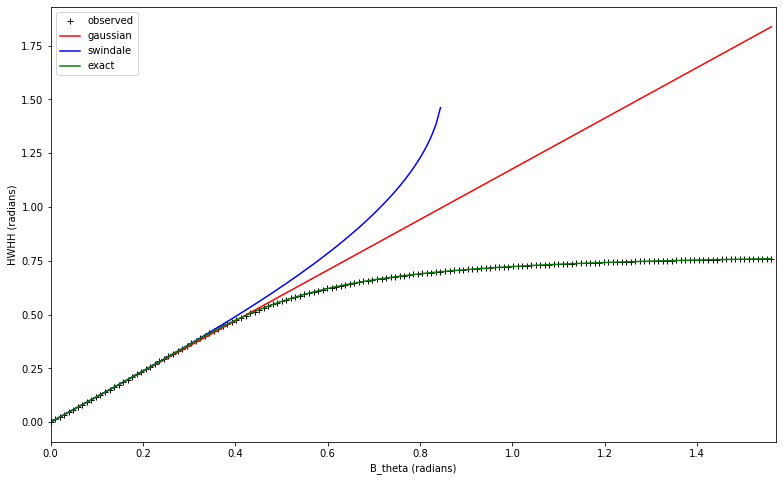Testing Grating

testing the orientation component¶

On this page, we concerntrate on the orientation variable and test the effect of changing the average orientation as well as the bandwidth.

In :
import numpy as np
np.set_printoptions(precision=3, suppress=True)
import pylab
import matplotlib.pyplot as plt
%matplotlib inline
In :
import MotionClouds as mc
import os
fx, fy, ft = mc.get_grids(mc.N_X, mc.N_Y, mc.N_frame)
help(mc.envelope_orientation)
Help on function envelope_orientation in module MotionClouds:

envelope_orientation(fx, fy, ft, theta=0.0, B_theta=0.19634954084936207)
Returns the orientation envelope:
We use a von-Mises distribution on the orientation:
- mean orientation is theta (in radians),
- B_theta is the bandwidth (in radians). It is equal to the standard deviation of the Gaussian
envelope which approximate the distribution for low bandwidths. The Half-Width at Half Height is
given by approximately np.sqrt(2*B_theta_**2*np.log(2)).

Run 'testing-grating.py' notebook to see the effect of changing theta and B_theta, see
http://motionclouds.invibe.net/posts/testing-grating.html

In :
name = 'grating'
#initialize
fx, fy, ft = mc.get_grids(mc.N_X, mc.N_Y, mc.N_frame)

z = mc.envelope_gabor(fx, fy, ft)
mc.figures(z, name, vext='.gif')
mc.figures(z, name)
mc.in_show_video(name)In :
# explore parameters
for sigma_div in [1, 2, 3, 5, 8, 13 ]:
name_ = name + '-largeband-B_theta-pi-over-' + str(sigma_div).replace('.', '_')
z = mc.envelope_gabor(fx, fy, ft, B_theta=np.pi/sigma_div)
mc.figures(z, name_)
mc.in_show_video(name_)In :
for div in [1, 2, 4, 3, 5, 8, 13, 20, 30]:
name_ = name + '-theta-pi-over-' + str(div).replace('.', '_')
z = mc.envelope_gabor(fx, fy, ft, theta=np.pi/div)
mc.figures(z, name_)
mc.in_show_video(name_)In :
V_X = 1.0
for sigma_div in [1, 2, 3, 5, 8, 13 ]:
name_ = name + '-B_theta-pi-over-' + str(sigma_div).replace('.', '_') + '-V_X-' + str(V_X).replace('.', '_')
z = mc.envelope_gabor(fx, fy, ft, V_X=V_X, B_theta=np.pi/sigma_div)
mc.figures(z, name_)
mc.in_show_video(name_)In :
for B_sf in [0.05, 0.1, 0.15, 0.2, 0.3, 0.4, 0.8]:
name_ = name + '-B_sf-' + str(B_sf).replace('.', '_')
z = mc.envelope_gabor(fx, fy, ft, B_sf=B_sf)
mc.figures(z, name_)
mc.in_show_video(name_)tuning the bandwidth¶

In the MotionClouds script, we define the orientation envelope based on a VonMises distribution:

In :
def vonmises(th, theta, kappa, norm=True):
if kappa==0:
env = np.ones_like(th)
elif kappa==np.inf:
env = np.zeros_like(th)
env[np.argmin(th < theta)] = 1.
else:
env = np.exp(kappa*(np.cos(2*(th-theta))-1))
if norm:
return env/env.sum()
else:
return env

This definition handles also the extreme cases when the bandwidth is infinite (in which case we return a flat distribution) of null (so we get a dirac). Note also that the von Mises distribution is on a continuous variable (orientation), while we use a discrete fourier transform. In particular, some care should be taken for very narrow bandwidths.

In :
N_theta = 16
theta, B_sf, B_V = np.pi/2, .1, .3
bins = 60
th = np.linspace(0, np.pi, bins, endpoint=False)
fig, ax = plt.subplots(1, 1, figsize=(13, 8))
B_theta_ = [np.pi/640, np.pi/32, np.pi/16, np.pi/8, np.pi/4, np.pi/2, np.inf]
for B_theta, color in zip(B_theta_, [plt.cm.hsv(h) for h in np.linspace(0, 1, len(B_theta_))]):
kappa = 1./4/B_theta**2
ax.plot(th, vonmises(th, theta, kappa), alpha=.6, color=color, lw=3)
ax.fill_between(th, 0, vonmises(th, theta, B_theta), alpha=.1, color=color)
_ = ax.set_xlim([0, np.pi])Getting everything peaking at one:

In :
N_theta = 16
theta, B_sf, B_V = np.pi/2, .1, .3
bins = 360
th = np.linspace(0, np.pi, bins, endpoint=False)
fig, ax = plt.subplots(1, 1, figsize=(13, 8))
for B_theta, color in zip(B_theta_, [plt.cm.hsv(h) for h in np.linspace(0, 1, len(B_theta_))]):
kappa = 1./4/B_theta**2
ax.plot(th, vonmises(th, theta, kappa, norm=False), alpha=.6, color=color, lw=3)
ax.fill_between(th, 0, vonmises(th, theta, kappa, norm=False), alpha=.1, color=color)
_ = ax.set_xlim([0, np.pi])Let's check that the HWHH (half-width at half-height) is given by the bandwidth parameter B_theta. Note that for high values of B_theta, the minimum of the distribution may be higher than 1/2 and that this decriptive value may be ill-defined (see for instance Swindale, N. V. (1998). Orientation tuning curves: empirical description and estimation of parameters. Biological Cybernetics, 78(1):45-56 - PDF). We will use the broader definition by using the fully normalized von mises that peaks at one and with a minimum at 0 : $$M(\theta) = \frac {\exp( \kappa \cdot cos(2\theta)) - \exp( -\kappa) }{\exp(\kappa) - \exp( -\kappa) }$$ The different expression for the HWHH are for the Gaussian approximation: $$\theta_{HWHH} = \sqrt{2\ln(2)} \cdot \kappa$$ for the non-normalized von-Mises pdf (as in Swindale): $$\theta_{HWHH} = \frac 1 2 \cdot \arccos (1 + \frac{ \ln( \frac 1 2 )}{\kappa})$$for the normalized (exact) solution: $$\theta_{HWHH} = \frac 1 2 \cdot \arccos (1 + \frac{\ln(\frac{1+e^{-2\kappa})}{2}}{\kappa})$$

In :
N_theta = 160
theta, B_sf, B_V = 0, .1, .3
B_theta_ = np.linspace(0, np.pi/2, N_theta, endpoint=False)
bins = 3600
th = np.linspace(0, np.pi, bins, endpoint=False)
HWHH = np.zeros(N_theta)
for _, B_theta in enumerate(B_theta_):
kappa = 1./4/B_theta**2
dist = vonmises(th, theta, kappa)
dist = (dist - dist.min())/(dist.max() - dist.min())
ind_HWHH = np.argmax(dist<.5)
HWHH[_] = th[ind_HWHH]

fig, ax = plt.subplots(1, 1, figsize=(13, 8))
ax.plot(B_theta_, HWHH, 'k+', label='observed')
ax.plot(B_theta_, B_theta_*np.sqrt(2*np.log(2)), 'r', label='gaussian')
k= 1./4/B_theta_**2
ax.plot(B_theta_, .5*np.arccos(1-np.log(2)/k), 'b', label='swindale')
ax.plot(B_theta_, .5*np.arccos(1+ np.log((1+np.exp(-2*k))/2)/k), 'g', label='exact')